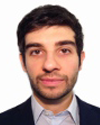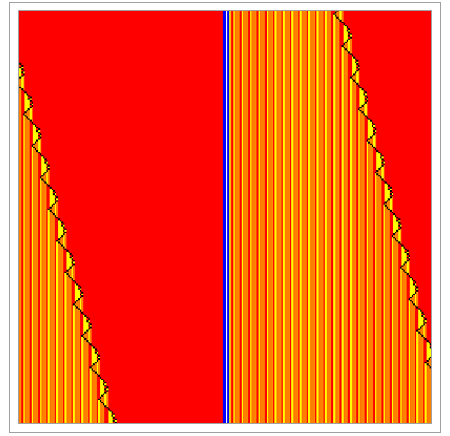#Wolfram Summer School

# Alumni# Giacomo Di Gesú

Summer School

Class of 2013

## Bio

Giacomo Di Gesú is currently a Postdoc in the Department of Mathematics at the University “La Sapienza” in Rome.

In his research, he has considered problems in asymptotic analysis of Markov processes arising in the field of statistical mechanics. He worked in particular on quantitative results to improve the mathematical understanding of so-called metastability phenomena. These show up in the dynamical behavior of a large variety of complex real-world systems and can be mimicked using simple mathematical models.

In his PhD thesis “Semiclassical Analysis of Discrete Witten Laplacians,” an analytic approach to determine critical metastable transition times in a discrete space setting is developed. Hereby some classical continuous space notions and techniques, originating in differential geometry and quantum mechanics, are recast to fit the discrete space models.

## Project: Tracing Mathematical Errors

In my project I want to consider the following problem: given a wrong answer to a certain mathematical question, can we construct a reasonable model that automatically traces to the source of the error by just knowing the wrong answer?

The world of wrong solutions to a question is of course more complex and variegated than the world of correct solutions. As Tolstoy puts it in War and Peace, “Happy families are all alike; every unhappy family is unhappy in its own way.”

To start with, I will consider only simple arithmetic and algebra school-level questions. The first step is to construct a tree-like model, which statistically describes the (possibly wrong) ways to solve the exercise. The parameters of the model will be chosen according to the available data on distributions of common errors. The next step is to devise an algorithm, taking as input the question and the wrong answer, and producing output suggestions on where to find the source of the error.

## Favorite Four-Color, Four State Turing Machine

Rule 1284073402777710443911• 21st Annual Wolfram Summer School
• Bentley University, Waltham, MA, USA
• June 25–July 15, 2023